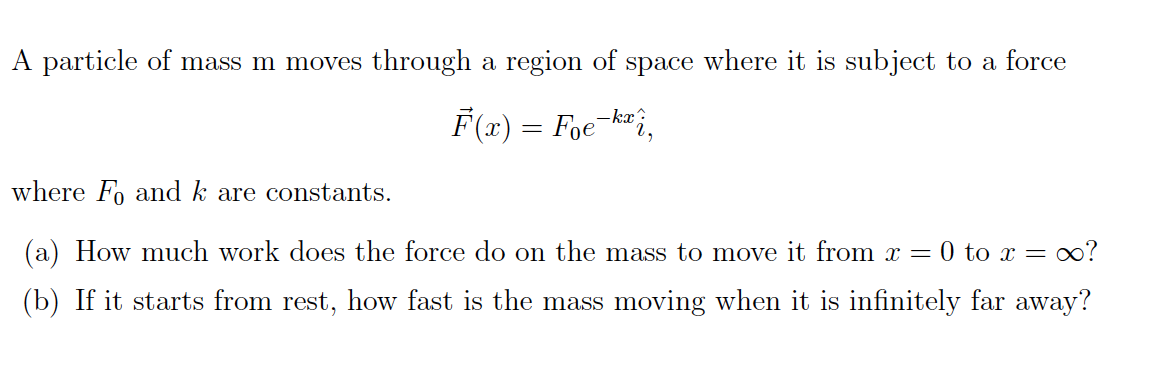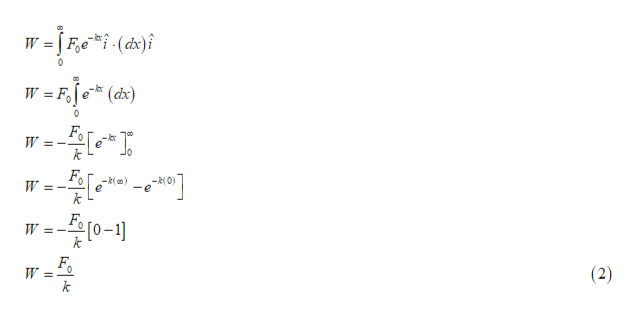A particle of mass m movesthrough a region of space where it is subjectforceto aF(x) = Foe-kx?where F and k are constants(a) How much work does the force do on theoo?mass to move it fromT = 0 to x =(b) If it starts from rest, how fast is the massmoving when it is infinitely far away?

Questionhelp_outlineImage TranscriptioncloseA particle of mass m moves through a region of space where it is subject force to a F(x) = Foe -kx? where F and k are constants (a) How much work does the force do on the oo? mass to move it from T = 0 to x = (b) If it starts from rest, how fast is the mass moving when it is infinitely far away? fullscreen
Step 1

(a)

According to the question force acts on particle along positive x direction and work done by given force is given by:

Step 2

Substitute limits given in question and value of force into equation (1) we get,help_outlineImage Transcriptionclose- F - kr e W F -( a) e -0-11 k (2) k fullscreen
Step 3

(b)

According to work energy theorem work done by all forces are equ...

Want to see the full answer?

See Solution

Want to see this answer and more?

Our solutions are written by experts, many with advanced degrees, and available 24/7

See Solution
Tagged in

Physics